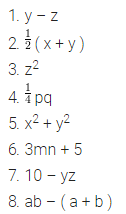# Get the algebraic expressions in the following cases using variables,

Get the algebraic expressions in the following cases using variables, constants and arithmetic operations.

1. Subtraction of z from y.
2. One-half of the sum of numbers x and y.
3. The number z multiplied by itself
4. One-fourth of the product of numbers p and q.
5. Numbers x and y both squared and added.
6. Number 5 added to three times the product of numbers m and n.
7. Product of numbers y and z subtracted from 10.
8. Sum of numbers a and b subtracted from their product.